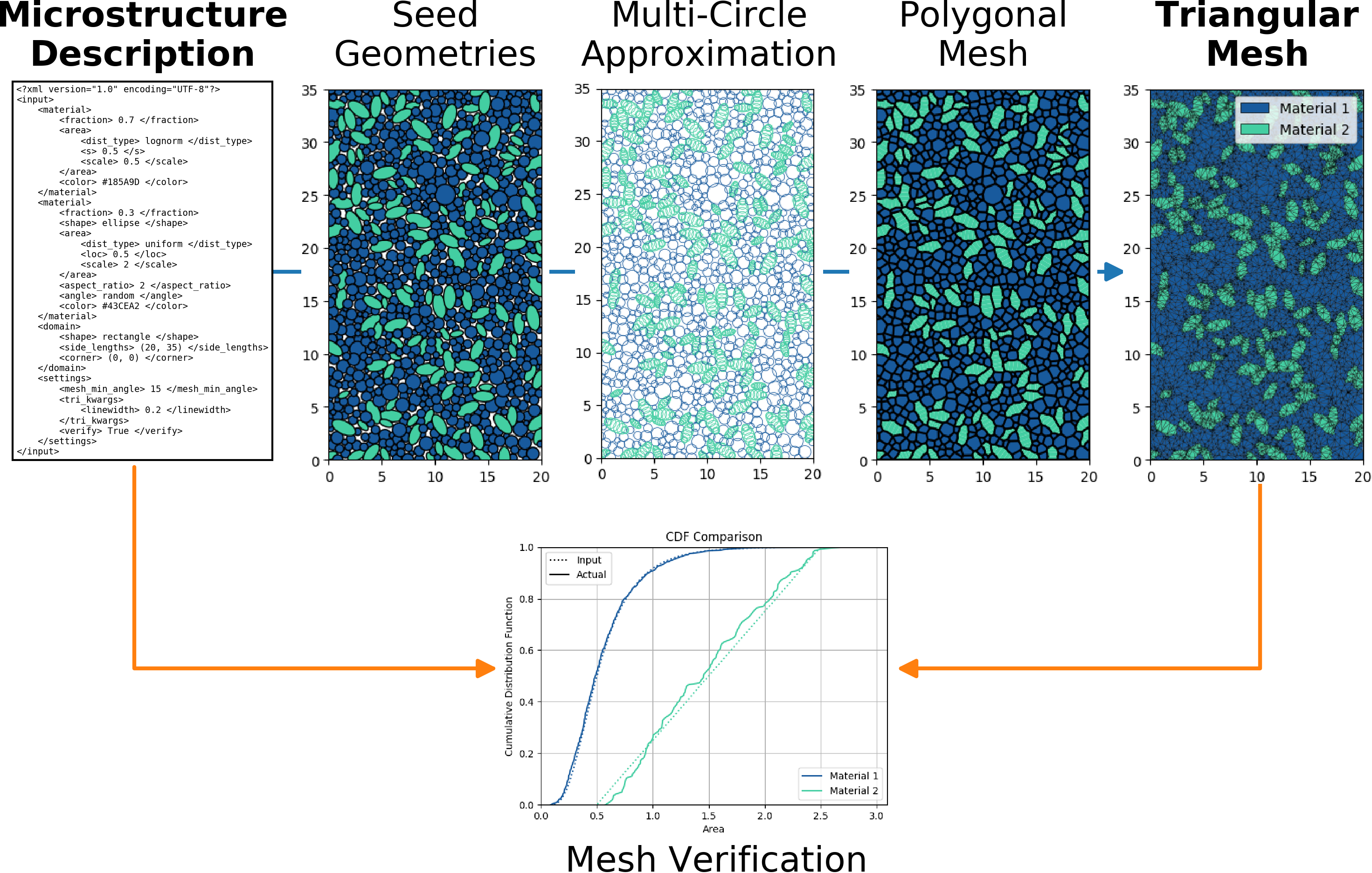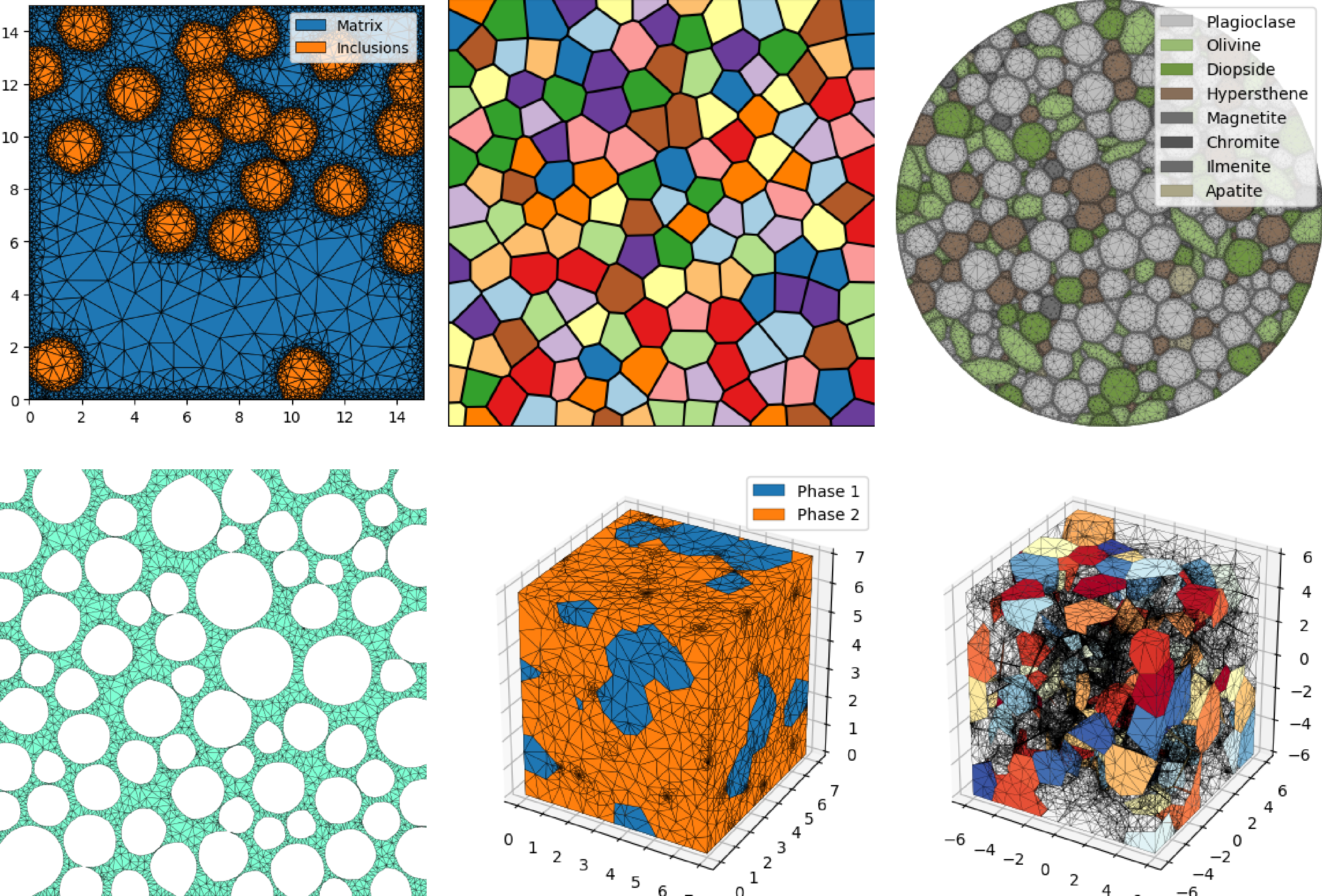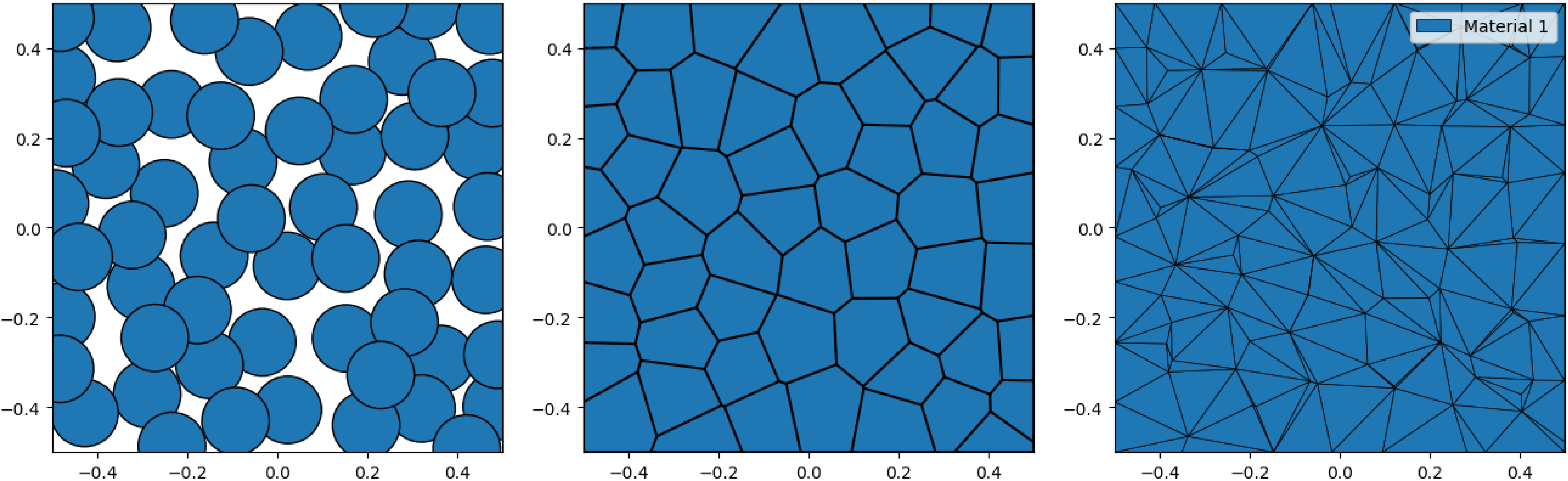MicroStructPy - Microstructure Mesh Generation in Python¶RepositoryDocumentationPyPI

MicroStructPy is a microstructure mesh generator written in Python. Features of MicroStructPy include:

• 2D and 3D microstructures

• Grain size, shape, orientation, and position control

• Polycrystals, amorphous phases, and voids

• Mesh verification

• Visualizations

• Output to common file formats

• Customizable workflowFig. 1 The MicroStructPy workflow.

MicroStructPy reads an XML file describing the composition of the microstructure, grain size distributions, and the size of the domain. Through the steps shown in the flowchart, this description is converted into a triangular/tetrahedral mesh. MicroStructPy can also compare the statistical distributions from the input file with those from the output mesh.

Examples¶

These images were created using MicroStructPy. For more examples, see the Examples section.Fig. 2 Examples created using MicroStructPy.

Quick Start¶

pip install microstructpy

If there is an error with the install, try pip install pybind11 first, then install MicroStructPy. This will create a command line executable and python package both named microstructpy. To use the command line interface, create a file called input.xml and copy this into it:

<?xml version="1.0" encoding="UTF-8"?>
<input>
<material>
<shape> circle </shape>
<size> 0.15 </size>
</material>

<domain>
<shape> square </shape>
</domain>
</input>

Next, run the file from the command line:

microstructpy input.xml

This will produce three text files and three image files: seeds.txt, polymesh.txt, trimesh.txt, seeds.png, polymesh.png, and trimesh.png. The text files contain all of the data related to the seed geometries and meshes. The image files contain:Fig. 3 The output plots are: 1) seed geometries, 2) polygonal mesh, and 3) triangular mesh.

The same results can be produced using this script:

import matplotlib.pyplot as plt
import microstructpy as msp

phase = {'shape': 'circle', 'size': 0.15}
domain = msp.geometry.Square()

# Unpositioned list of seeds
seeds = msp.seeding.SeedList.from_info(phase, domain.area)

# Position seeds in domain
seeds.position(domain)

# Create polygonal mesh
polygon_mesh = msp.meshing.PolyMesh.from_seeds(seeds, domain)

# Create triangular mesh
triangle_mesh = msp.meshing.TriMesh.from_polymesh(polygon_mesh)

# Plot outputs
for output in [seeds, polygon_mesh, triangle_mesh]:
plt.figure()
output.plot(edgecolor='k')
plt.axis('image')
plt.axis([-0.5, 0.5, -0.5, 0.5])
plt.show()